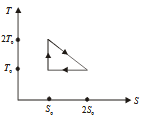#### The temperature­- entropy diagram of a reversible engine cycle is given in the figure. Its efficiency isOption 1)Option 2)Option 3)Option 4)As we learnt in

Entropy -

It is a measure of disorder of molecular motion of a system.

- wherein

Greater is disorder greater is entropyand

Efficiency of a cyclic process -- wherein

Gross heat implied only part of heat absorbed.

The efficiency of cycle is:EfficiencyOption 1)This option is correct.

Option 2)This option is incorrect.

Option 3)This option is incorrect.

Option 4)This option is incorrect.

#### prateek